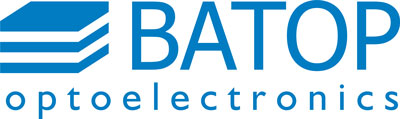#### SOC - Saturable Output coupler λ = 1064 nm

• see flyer "SOC - saturable output coupler" (pdf)

SOC order information:
Part-No description: SOC-λ-A-T-τ-x
• λ - laser wavelength
• A - low intensity absorption
• T - low intensity transmittance
• τ - absorber relaxation time
• x - mounting options
Product list SOC, λ = 1064 nm

• Wavelength λ = 1064 nm
• Absorptance A = 1 %
• Transmittance T = 1 %
• Relaxation time τ = 5 ps
Data sheet (pdf)
Mounting & price table
Order form

• Wavelength λ = 1064 nm
• Absorptance A = 1.8 %
• Transmittance T = 2.2 %
• Relaxation time τ = 1 ps
Data sheet (pdf)
Mounting & price table
Order form

• Wavelength λ = 1064 nm
• Absorptance A = 2 %
• Transmittance T = 0.3 %
• Relaxation time τ = 1 ps
Data sheet (pdf)
Mounting & price table
Order form

• Wavelength λ = 1064 nm
• Absorptance A = 2.7 %
• Transmittance T = 3.2 %
• Relaxation time τ = 1 ps
Data sheet (pdf)
Mounting & price table
Order form

• Wavelength λ = 1064 nm
• Absorptance A = 4 %
• Transmittance T = 0.5 %
• Relaxation time τ = 1 ps
Data sheet (pdf)
Mounting & price table
Order form

• Wavelength λ = 1064 nm
• Absorptance A = 7 %
• Transmittance T = 0.7 %
• Relaxation time τ = 1 ps
Data sheet (pdf)
Mounting & price table
Order form

• Wavelength λ = 1064 nm
• Absorptance A = 8 %
• Transmittance T = 6 %
• Relaxation time τ = 1 ps
Data sheet (pdf)
Mounting & price table
Order form

• Wavelength λ = 1064 nm
• Absorptance A = 8 %
• Transmittance T = 8 %
• Relaxation time τ = 15 ps
Data sheet (pdf)
Mounting & price table
Order form

• Wavelength λ = 1064 nm
• Absorptance A = 9 %
• Transmittance T = 13 %
• Relaxation time τ = 17 ps
Data sheet (pdf)
Mounting & price table
Order form

• Wavelength λ = 1064 nm
• Absorptance A = 13 %
• Transmittance T = 12 %
• Relaxation time τ = 15 ps
Data sheet (pdf)
Mounting & price table
Order form

• Wavelength λ = 1064 nm
• Absorptance A = 15 %
• Transmittance T = 23 %
• Relaxation time τ = 17 ps
Data sheet (pdf)
Mounting & price table
Order form

• Wavelength λ = 1064 nm
• Absorptance A = 21 %
• Transmittance T = 13 %
• Relaxation time τ = 20 ps
Data sheet (pdf)
Mounting & price table
Order form

• Wavelength λ = 1064 nm
• Absorptance A = 22 %
• Transmittance T = 19 %
• Relaxation time τ = 15 ps
Data sheet (pdf)
Mounting & price table
Order form

Mounting options x & price

• x = 5.0-0

• Single chip, unmounted
• Chip area 5.0 mm x 5.0 mm
• Chip thickness 625 µm
Data sheet (pdf)
• Price: 699.- €/pc

• x = 5.0-12.7g

• Chip area 5.0 mm x 5.0 mm
• Glued on a copper heat sink with 12.7 mm diameter and 4 mm center hole
Data sheet (pdf)
• Price: 786.- €/pc

• x = 5.0-25.4g

• Chip area 5.0 mm x 5.0 mm
• Glued on a copper heat sink with 25.4 mm diameter and 4 mm center hole
Data sheet (pdf)
• Price: 786.- €/pc

• x = FC

• SOC chip mounted on a single mode fiber cable with FC/PC connector
• free space output beam
Data sheet (pdf)
• Price: 976.- €/pc